# Thermodynamic potential facts for kids

Kids Encyclopedia Facts

In thermodynamics, thermodynamic potentials are parameters associated with a thermodynamic system and have the dimensions of energy. They are called "potentials" because in a sense, they describe the amount of potential energy in a thermodynamic system when it is subjected to certain constraints. The different potentials correspond to different constraints to which the system may be subjected. The four most common thermodynamic potentials are:

 Name Formula Natural variables Internal energy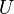$U\,$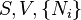$~~~~~S,V,\{N_i\}\,$ Helmholtz free energy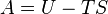$A=U-TS\,$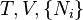$~~~~~T,V,\{N_i\}\,$ Enthalpy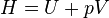$H=U+pV\,$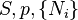$~~~~~S,p,\{N_i\}\,$ Gibbs free energy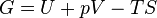$G=U+pV-TS\,$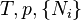$~~~~~T,p,\{N_i\}\,$

where T = temperature, S = entropy, p = pressure, V = volume. The Helmholtz free energy is often denoted by the symbol F, but the use of A is preferred by IUPAC (See Alberty, 2001).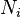$N_i$ is the number of particles of type i in the system. For the sake of completeness, the set of all$N_i$ are also included as natural variables, although they are sometimes ignored.

## Description and interpretation

Thermodynamic potentials are very useful when calculating the equilibrium results of a chemical reaction, or when measuring the properties of materials in a chemical reaction. The chemical reactions usually take place under some simple constraints such as constant pressure and temperature, or constant entropy and volume, and when this is true, there is a corresponding thermodynamic potential which comes into play. Just as in mechanics, the system will tend towards lower values of potential and at equilibrium, under these constraints, the potential will take on an unchanging minimum value. The thermodynamic potentials can also be used to estimate the total amount of energy available from a thermodynamic system under the appropriate constraint.Thermodynamic potential Facts for Kids. Kiddle Encyclopedia.